Previous: 6.2.1 Phase Space Distribution Up: 6.2 Quantum Mechanics in Next: 6.2.3 Operator-Theoretic Structure

## 6.2.2 Definition of Wigner Function

Among the several transforms of the wave function or in general of the density operator, that provide a phase space view of the quantum state, the Wigner function is the one that has found more applications, mainly in statistical mechanics, but also in quantum chemistry, molecular dynamics, scattering theory, or quantum optics.

The Wigner function is (up to a constant factor) the Weyl transform of the quantum-mechanical density operator. For a particle in one dimension it takes the form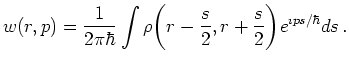(6.40)

Here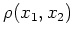denotes the von Neumann density function, i.e.,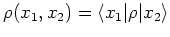(6.41)

with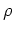the density operator.

This transformation can be conveniently split into two steps. For this we define: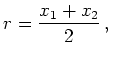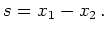(6.42)

We call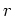the center of mass coordinate and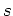the distance coordinate. These names should not be physically misleading as the von Neumann equation does not describe a two-particle problem but quantum correlations.

The inverse relation is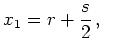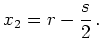(6.43)

Calculation of the Wigner distribution involves as a first step a change to new coordinatesand. This gives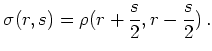(6.44)

We call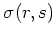the center of mass (com) distribution.

In a second step this is followed by a Fourier transformation with respect to.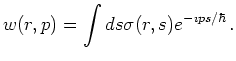(6.45)

An inverse Fourier transform gives the com distribution from the Wigner distribution: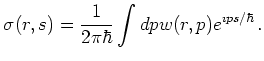(6.46)

Instead of the momentum coordinate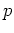it is possible to introduce the wave vector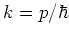which eliminates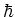from the transformation: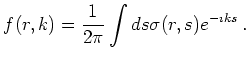(6.47)

Note that asis self-adjoint,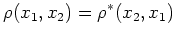we have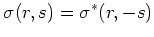and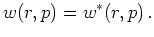So the Wigner distribution is real. However, in contrast to its classical analogon it can assume negative values. As position and momentum operators do not commute and hence cannot be measured together, this does not present an inconsistency.

The Wigner distribution possesses an important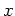-duality given by the alternative definition [FJP03]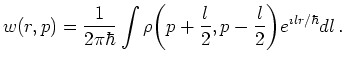(6.48)

Heredenotes the density function in momentum representation, that is: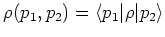where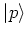denotes the eigenvector of the momentum operator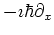with eigenvalue.